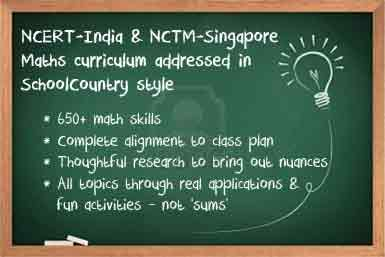Login/Register Math Worksheets, Activities, Games Helpline 099826-99306Complete curriculum and more

What is our Curriculum Plan
BrainX Math training program is completely aligned to the curriculum prescribed by NCERT-India and NCTM-Singapore The same guidelines are followed by schools.
We take these curriculum guidelines and then break it down in to over 650 Math Skills. For example,we have over 17 types of additions skills because for young students, vertical addition is different from horizontal addition, picture addition, word problems and so on. We address each skill systematically through our programs.
Below you will find a high level description of curriculum followed by BrainX program.
Objective of Class 1 BrainX Math program:
Develop an understanding of addition and subtraction strategies and knowledge of addition and subtraction facts. Work on whole number relationships including TENS and UNITS and composing and decomposing geometrical shapes
 Numbers Numbers notation and place value Cardinal and ordinal numbers Comparison and ordering Arithmetic Operations Addition of 1 and 2 digit numbers Subtraction of 1 and 2 digit numbers Multiplication tables when product is less than 40 Money and measures Sense of length Sense of mass Time Money Geometry Shapes Patterns
Start the full program for Class 1
Objective of Class 2 BrainX Math program:
Understand base-10 number system and place value concepts. Build quick recall of addition, subtraction and multiplication facts and develop fluency in up to 2 digit addition. Get practical feel of geometrical concepts and measurements
 Whole Numbers Numbers notation and place value up to THOUSANDS Number to words Comparison and ordering Arithmetic Operations Addition of two numbers up to 3 digits Subtraction of two numbers up to three digits Multiplication facts with 1, 2, 3, 4, 5 and 10 Division as reverse multiplication One step word problems for the three operations Geometry Knowledge of basic 2D shapes Recall of names of basic 3D shapes Money and measures Measures of length, mass and volume Estimation of length, mass and volume using metric units Addition and subtraction of length, mass and volume Read time from analogue clocks Addition and subtraction of money One step word problems involving length, mass, volume, time and money Statistics Pictures and graph using scale representations
Start the full program for Class 2
Objective of Class 3 BrainX Math program:
Developing an understanding of multiplication and division related facts. Building competence in complex addition and subtraction operations. Understand concept of fractions as part of a whole and gain insights in to properties of 2D shapes
 Numbers Number notation and place value - up to TEN THOUSAND Odd and even numbers Arithmetic Operations Addition of up to 4 digit numbers Subtraction of up to 4 digit numbers Multiplication tables up to 10 X 10 Multiplication and division by 1 digit numbers Word problems involving above operations using 2 steps Geometry Knowledge of 2D, 3D shapes Properties of 2D, 3D shapes Introduction to concept of angles Symmetry of shapes, mirror images Money and measures Standard numbers and measuring in compound units Addition and subtraction of length, mass, volume and time Addition and subtraction of money Word problems using 2 steps involving money and measures Perimeter of rectilinear figures Reading of digital and analog clocks Fractions Fractions as part of a whole Statistics Reading and interpretation of bar graphs
Start the full program for Class 3
Objective of Class 4 BrainX Math program:
Enable quick recall of multiplication facts and build proficiency with division. Develop an understanding of concept of area and perimeter. Develop proficiency with length, volume, weight, money and time.
 Numbers Number notation and place value up to LAKH / HUNDRED THOUSAND Approximation and estimation to nearest 10 and 100 Estimations involving addition, subtraction and multiplication Arithmetic operations Multiplication of up to two digit numbers Multiplication and division of money Word problems using 3 steps involving whole numbers and all four operations Geometry Perpendicular and parallel lines Angles in degrees Symmetry of shapes and mirror images Knowledge of different triangles and quadrilaterals Properties of a square and rectangle 2-D representation of a 3-d solid Factors and multiples Factors and multiples up to 100 Fractions Fractions as part of a whole Comparison of fractions Equivalence of fractions Money and Measures Multiplication and division of length, mass, volume and time Multiplication and division of money Volume of a cube/'cuboid and liquid Area and Perimeter of a square, rectangle and related figures Statistics Interpretation of tables, bar graphs Word Problems using data from bar graphs
Start the full program for Class 4099826-99306